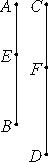# Proposition 11

If a whole is to a whole as a subtracted number is to a subtracted number, then the remainder is to the remainder as the whole is to the whole.

Let the whole AB be to the whole CD as AE subtracted is to CF subtracted.I say that the remainder EB is to the remainder FD as the whole AB is to the whole CD.

Since AB is to CD as AE is to CF, therefore AE is the same part or parts of CF as AB is of CD. Therefore the remainder EB is the same part or parts of FD that AB is of CD.

VII.Def.20

Therefore EB is to FD as AB is to CD.

Therefore, if a whole is to a whole as a subtracted number is to a subtracted number, then the remainder is to the remainder as the whole is to the whole.

Q.E.D.

## Guide

This proposition is the numerical analogue of proposition V.19 for general magnitudes. Symbolically,

if a : c = e : f, then a – e : c – f = a : c.

This proposition is used in IX.35.